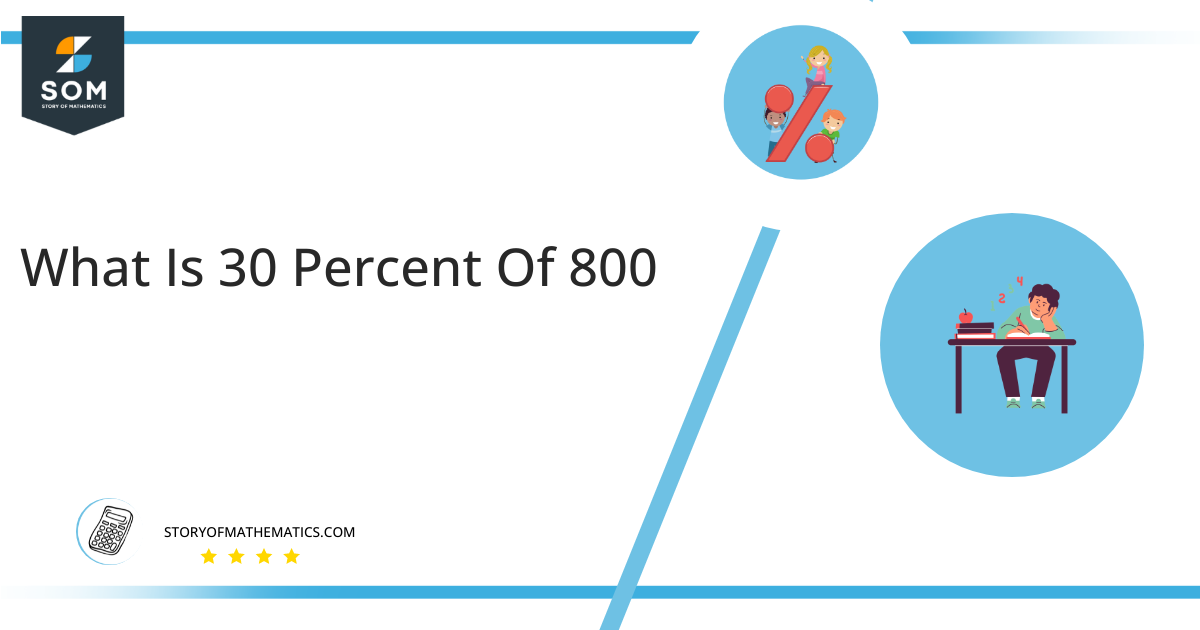# What Is 30 Percent of 800 + Solution With Free Steps30 percent of 800 is 240. To obtain this answer, we multiply 0.30 by 800.

Let’s suppose that you buy something from daraz and the cost of the item is 800 dollars. The delivery charge on the item is 30 percent of the price of the item from the seller. The price of the item is 800 dollars. So you need to pay 240 dollars as delivery charges as 30 percent of 800 is 240.

## What Is 30 Percent of 800?

30 percent of 800 is 240. To calculate this answer, we multiply 0.30 by 800.

To arrive at the same conclusion, one can also multiply the fraction 30/100 by the full number 800 to do the calculation. This would result in an output of 240, which is what was sought, or a result that is comparable.

## How to calculate 30 percent of 800?

The value that equals 30% of 800 can be calculated by using the following mathematical steps in the correct order.### Step 1

The mathematical expression of 30% of 800 is:

30 percent of 800 = 30% x 800

### Step 2

Substitute the percentage sign by 1/100 in the above equation:

30 percent of 800 = ( 30 x 1/100 ) x 800

### Step 3

Changing the order of the (30 x 1/100) x 800 results in:

30 percent of 800 = ( 30 x 800 ) / 100

### Step 4

Multiplying of 30 with 800:

30 percent of 800 = ( 24,000 ) / 100

### Step 5

Dividing 24,000 by 100:

30 percent of 800 = 240

30 percent of 800 equals 240, according to the preceding formula.

By expressing the data in a visual style, the below pie chart will help us understand that 30% of 800 is comparable to 240.Figure 1: The above pie chart shows 30 percent of 800.

The portion of figure shown in blue above is 30% of 800, which is 240. The red region represents 80 percent of 800, which is equivalent to 560.

According to its definition, the % scale is a special scale in which all values of a given amount are converted to a scale running from 0 to one hundred. When comparing different things, percentages are really helpful.

All the Mathematical drawings/images are created using GeoGebra.